# [Processing × Java] How to use the loop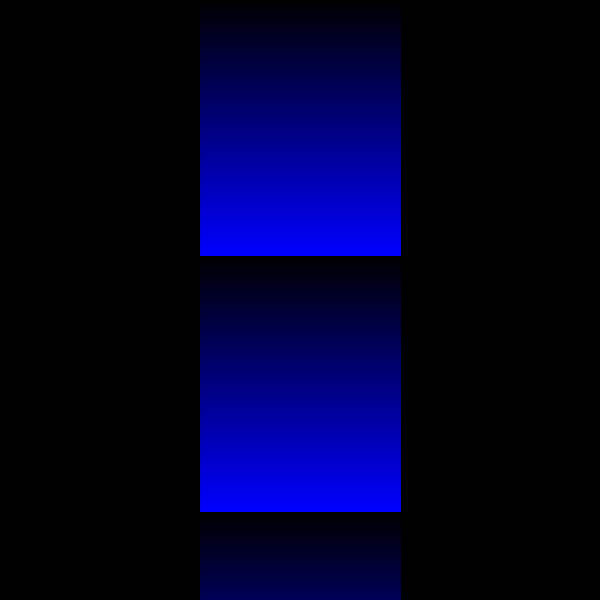2. Example of a program using a loop

# 0. What to use the loop for

** Used to organize repetitive processes **. The program below is a program for efficiently finding the sum of numbers from ** 1 to 10 **.

#### `loop00.java`

``````
//The variable sum is the sum you want to find
int sum = 0;

//Add i by 1 from 1 to 10.
for(int i = 1;i < 11;i++){
//Sum(new) = Sum(Old) + i
sum = sum + i;
}

//Display the sum on the console.
println(sum);
``````
``````55
``````
i sum sum + i
1 1 0 + 1
2 3 1 + 2
3 6 3 + 3
4 10 6 + 4
5 15 10 + 5
6 21 15 + 6
7 28 21 + 7
8 36 28 + 8
9 45 36 + 9
10 55 45 + 10

Point　: In order to use loops, you have to find ** common parts ** (property / law) among similar repetitive actions! In other words, you need to determine ** which part to repeat **.

## for loop

A for loop is one of the ways to create a loop. It's the same as a while loop, but it's more compact and easier to understand, so I'll introduce it here.

#### `loop01.java`

``````
//Determine the size of the screen.
size(500,250);
//Place the lines every 20 px.
for(int i = 0;i < width;i += 20){
line(i,0,i,height);
}
``````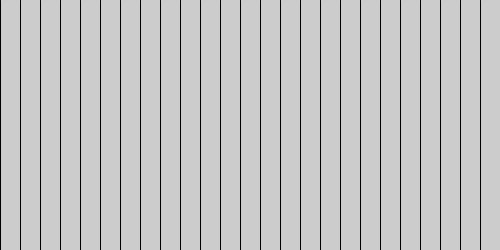** Point **: A program that draws a straight line drawn from (i, 0) to (i, height) every 20px within the range of i <width.

#### ○ ** For loop execution order ** (For example, in this program ...)

What is the value of i? : 0! ↓ Does the value of i (= 0) satisfy i <width? : True! ↓ Execution of processing ↓ i + = 20! ↓ What is the value of i? : 20! : : What is the value of i? : 500! ↓ Does the value of i (= 500) satisfy i <width? : False! (End of loop)

** Point **: i + = 20 is an abbreviation for i = i + 20.

#### ○ Image of for loop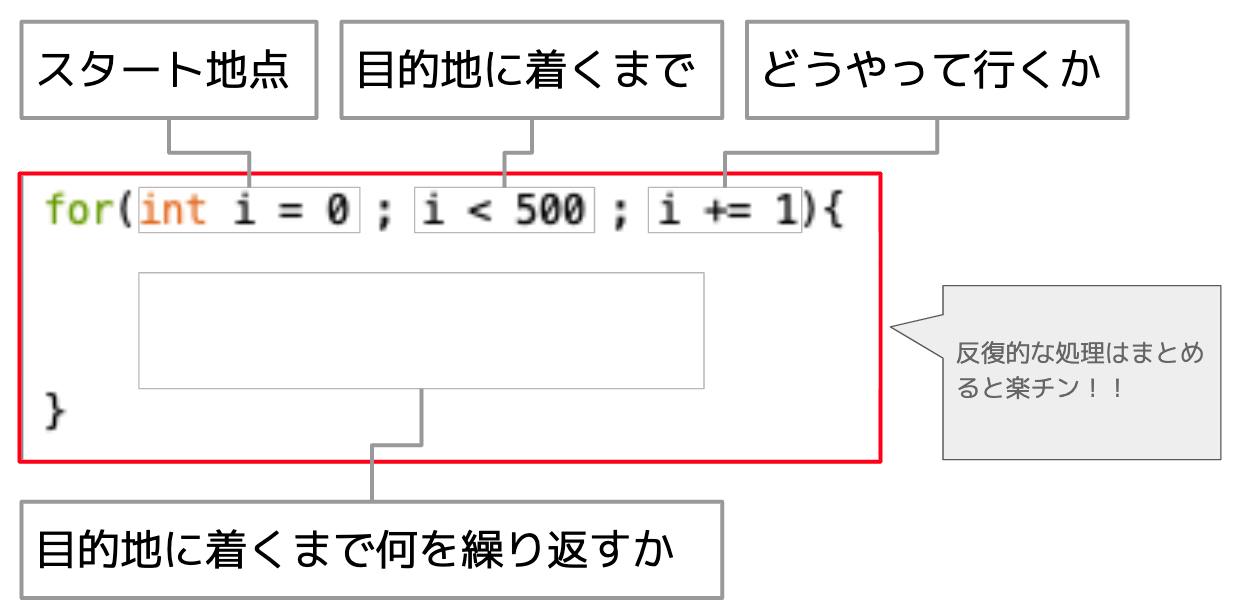** Point **: ** It may be close to that feeling of choosing sweets for an excursion so that it fits in 300 yen ...

# 2. Example of a program using for loop

#### `variable02.java`

``````
//Determine the size of the screen
size(500,500);
//Determine the background color
background(0);

//i <While satisfying the width, increase i by 10.
for(int i = 0;i < width;i += 10){
//Determine the color of the line
stroke(53,183,193);
//(0,i)From(i,height)Draw a line
line(0,i,i,height);
}
``````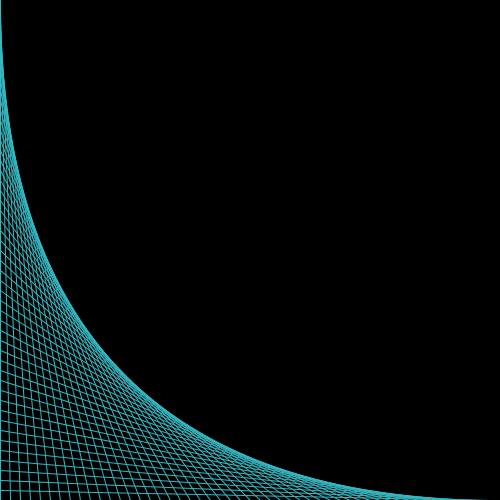** Point **: Processing coordinates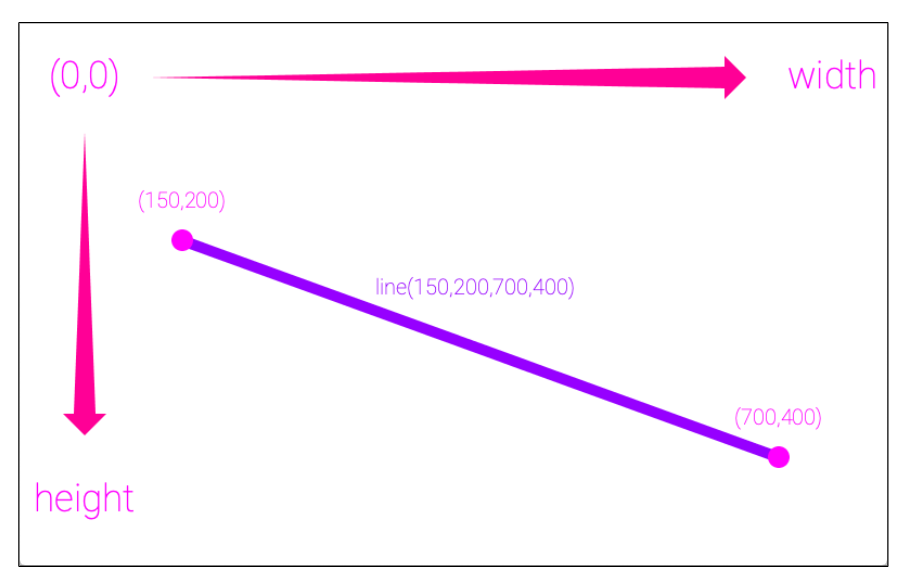The feature is that the value of y increases toward the bottom of the screen.

#### `variable03.java`

``````
//Determine the size of the screen
size(500,500);
//Determine the background color
background(0);

//i <While satisfying the width, increase i by 1.(Repeat 500 times)
for(int i = 0;i < width;i += 1){
//Determine the color of the line
stroke(#640915);
//Draw a line from a random point to a random point
line(random(500),random(500),random(500),random(500));
}
``````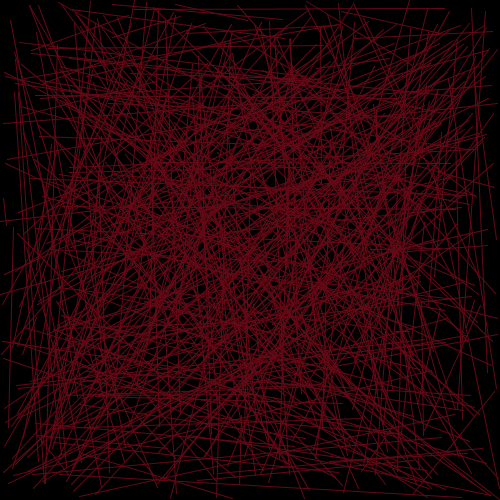Point　:　random(min,max); Returns (outputs) a random value in the range min to max. min can be omitted.

** Point **: Repeat the loop 500 times until i becomes 0,1,2, .... 498,499.

** Point **: There are other ways to select colors other than (R, G, B). [Tools]-> [Select Color]-> Select your favorite color from the menu bar

#### ◯ Loop and animation

It is effective to use draw () to create an animation.

#### `variable03.java`

``````
//Define your own variable s that represents the size of the circle
float s = 0;

//Repeat only once
void setup(){
size(600,600);
//The default is 60 frames per second
frameRate(10);
background(0);
}

//infinite loop
void draw(){
//Increase the size by 1
s += 1;
//Color specification
fill(random(255),0,255,50);
noStroke();
//Place circles in random places
ellipse(random(width),random(height),s,s);

//If the size is larger than 45, it will be executed
if (s > 45) {
//Return size to 0
s = 0;
}

}
``````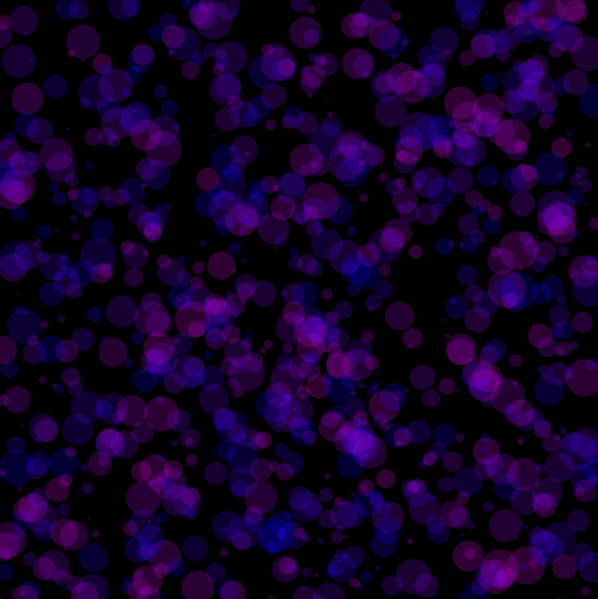# Finally

Thank you for reading. We appreciate your opinions and suggestions in order to make the article even better.